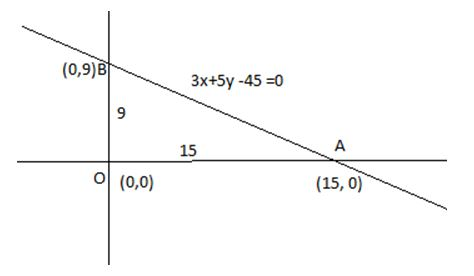# Let T be the triangle formed by the straight line | Quantitative Aptitude – CAT Modern Math – Set theory

## CAT 2019 – Slot -1 - Question 4 - Let T be the triangle formed by the straight line

Q. 4: Let T be the triangle formed by the straight line 3x + 5y – 45 = 0 and the coordinate axes. Let the circumcircle of T have radius of length L, measured in the same unit as the coordinate axes. Then, the integer closest to L is

given eq of straight line is 3x + 5y – 45 = 0,

the line cuts x axis at

Put y = 0, 3x – 45 = 0 or x = 15 , so (15, 0)

Put x = 0, 5y – 45= 0 ,or y = 9

Thus the line cut y –axis at (0,9)Thus we can say OAB is the triangle T which is right angled triangle so AB will be dia of triangle.

Diameter AB = (9^2 + 15^2)^1/2 = (81+225)^1/2 = (306)^1/2 = 17.54 (approx)

So radius = 17.54/2 = 9 (approx)

### Past Year Question Paper & SolutionsCounselling Session
By IIM Mentor

#### Free Material Area